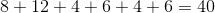ISEE Lower Level Quantitative : Parallelograms

Example Questions

← Previous 1 3 4 5 6 7 8 9 22 23

Example Question #1 : Parallelograms

Ted drew the parallelogram shown below.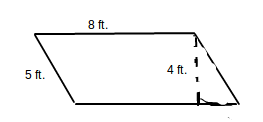What is the perimeter of the parallelogram?

40 feet

26 feet

17 feet

12 feet

13 feet

26 feet

Explanation:

The perimeter of a parallelogram can be found by summing the lengths of each of the sides.  In a parallelogram, opposite sides have the same length.  Therefore, you should use the equation 8 + 8 + 5 + 5 = P.  The perimeter of the parallelogram is 26 feet.

Example Question #2 : Parallelograms

Guillermo drew the parallelogram below.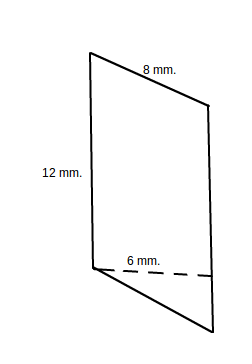What is the perimeter of the parallelogram?

42 millimeters

40 millimeters

20 millimeters

26 millimeters

72 millimeters

40 millimeters

Explanation:

The perimeter of a parallelogram can be found by summing the lengths of each of the sides.  In a parallelogram, opposite sides have the same length.  Therefore, you should use the equation 8 + 8 + 12 + 12 = P.  The perimeter of the parallelogram is 40 feet.

Example Question #13 : Plane Geometry

Find the perimeter of the following parallelogram.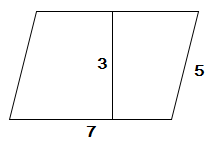Explanation:

We plug in our values into the equation for the perimeter: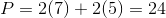Example Question #14 : Plane Geometry

Find the perimeter of the following parallelogram.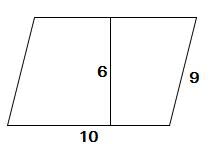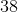Explanation:

We plug in our values into the equation for the perimeter: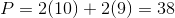Example Question #15 : Plane Geometry

Find the perimeter of the following parallelogram.Explanation:

We plug in our values into the equation for the perimeter:Example Question #16 : Plane Geometry

What is the perimeter of the polygon below?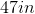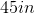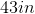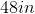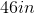Explanation:

To find the area of a perimeter, we add all of the side lengths together.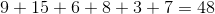Example Question #61 : Geometric Measurement: Recognizing Perimeter

What is the perimeter of the polygon below?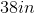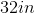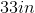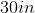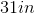Explanation:

To find the area of a perimeter, we add all of the side lengths together.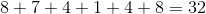Example Question #18 : Plane Geometry

What is the perimeter of the polygon below?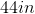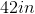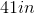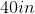Explanation:

To find the area of a perimeter, we add all of the side lengths together.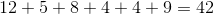Example Question #64 : Geometry

What is the perimeter of the polygon below?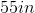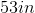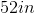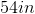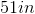Explanation:

To find the area of a perimeter, we add all of the side lengths together.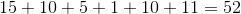Example Question #65 : Geometry

What is the perimeter of the polygon below?